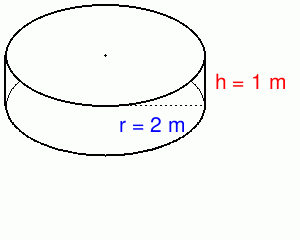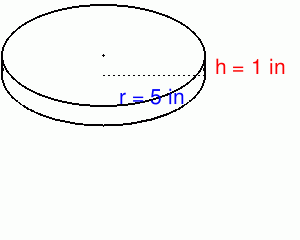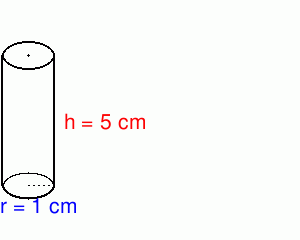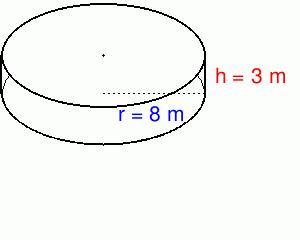# Math Practice Topic: Cylinders

 Description: Find the volumes of cylinders. Adaptive Learning Progression: More problems, higher difficulty. Start using MathScore for free

## Sample Levels (out of 6)

Find the surface area and volume. Answer with proper units like m2 and m3.

 1Surface Area = Volume =

Find the surface area and volume. Answer with proper units like m2 and m3.

 1Surface Area = Volume = 2Surface Area = Volume = 3Surface Area = Volume =